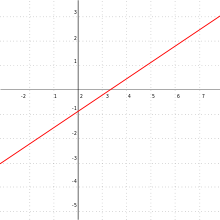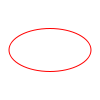# Plane curve

In mathematics, a plane curve is a curve in a plane that may be either a Euclidean plane, an affine plane or a projective plane. The most frequently studied cases are smooth plane curves (including piecewise smooth plane curves), and algebraic plane curves.

## Smooth plane curve

A smooth plane curve is a curve in a real Euclidean plane R2 and is a one-dimensional smooth manifold. This means that a smooth plane curve is a plane curve which "locally looks like a line", in the sense that near every point, it may be mapped to a line by a smooth function. Equivalently, a smooth plane curve can be given locally by an equation f(x, y) = 0, where f : R2R is a smooth function, and the partial derivatives f/x and f/y are never both 0 at a point of the curve.

## Algebraic plane curve

An algebraic plane curve is a curve in an affine or projective plane given by one polynomial equation f(x, y) = 0 (or F(x, y, z) = 0, where F is a homogeneous polynomial, in the projective case.)

Algebraic curves were studied extensively since the 18th century.

Every algebraic plane curve has a degree, the degree of the defining equation, which is equal, in case of an algebraically closed field, to the number of intersections of the curve with a line in general position. For example, the circle given by the equation x2 + y2 = 1 has degree 2.

The non-singular plane algebraic curves of degree 2 are called conic sections, and their projective completion are all isomorphic to the projective completion of the circle x2 + y2 = 1 (that is the projective curve of equation x2 + y2 - z2= 0). The plane curves of degree 3 are called cubic plane curves and, if they are non-singular, elliptic curves. Those of degree four are called quartic plane curves.

## Examples

Name Implicit equation Parametric equation As a function graph
Straight lineCircleParabolaEllipseHyperbola